Pandas Dataframe Examples: String Functions

# Pandas Dataframe Examples: String Functions

Last updated:

WIP Alert This is a work in progress. Current information is correct but more content may be added in the future.

View all examples on this jupyter notebook

If you call .str on a Series object that contains string objects, you get to call string methods on all Series elements.

## Select by partial string

Set regex=False for better performance

To filter rows by partial string, use <column>.str.contains():

import pandas as pd

df = pd.DataFrame({
'name': ['alice smith','bob jones','charlie joneson','daisy white'],
'age': [25,20,30,35]
})

df[df['name'].str.contains('jones',regex=False)]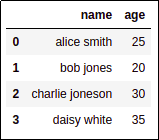Original dataframe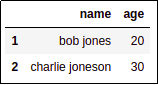After applyting filter: .str.contains('jones')

## Select like

Use Select by partial string above

## Select by regular expression

As before, to filter rows where the text matches a regular expression, just use .str.contains():

import pandas as pd

df = pd.DataFrame({
'name': ['alice smith','bob jones','charlie joneson','daisy white'],
'age': [25,20,30,35]
})

# names starting with 'b' or 'd'
df[df['name'].str.contains('^b|d')]Original dataframe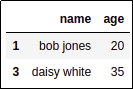Rows whose name column starts with
either a 'b' or a 'd'

## Concatenate string columns

Just use the + sign:

import pandas as pd

df = pd.DataFrame({
'first_name': ['alice','bob','charlie','daisy'],
'last_name':['smith','jones','joneson','white'],
'age': [25,20,30,35]
})

# just add the two columns
df['full_name'] = df['first_name'] + df['last_name']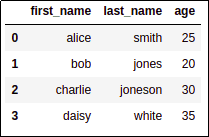Original dataframe with two separate columnsAFTER: columns concatenated. See below for how to

You can also add a simple string (whitespace) in between the columns; Pandas knows it should propagate that string to all rows:

df['full_name'] = df['first_name'] + ' ' + df['last_name']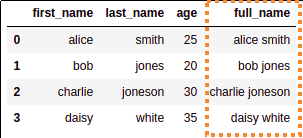Alternative version: You can also add
simple whitespace in between the two
columns so that the names are correct.

## Split string column

In order to split a string column into multiple columns, do the following:

• 1) Create a function that takes a string and returns a series with the columns you want

• 2) Use apply() on the original dataframe

• 3) Concatenate the created columns onto the original dataframe

import pandas as pd

df = pd.DataFrame({
'name': ['alice smith','bob jones','charlie joneson','daisy white'],
'age': [25,20,30,35]
})

# a function that takes the value and returns
# a series with as many columns as you want
def split_name(name):
first_name, last_name = name.split(' ')

return pd.Series({
'first_name': first_name,
'last_name': last_name
})

# df_new has the new columns
df_new = df['name'].apply(split_name)

# append the columns to the original dataframe
df_final = pd.concat([df,df_new],axis=1)Original dataframe has a single column
with the full name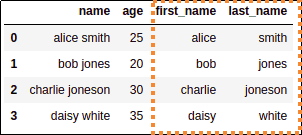Two new columns were created
by splitting full_name into two